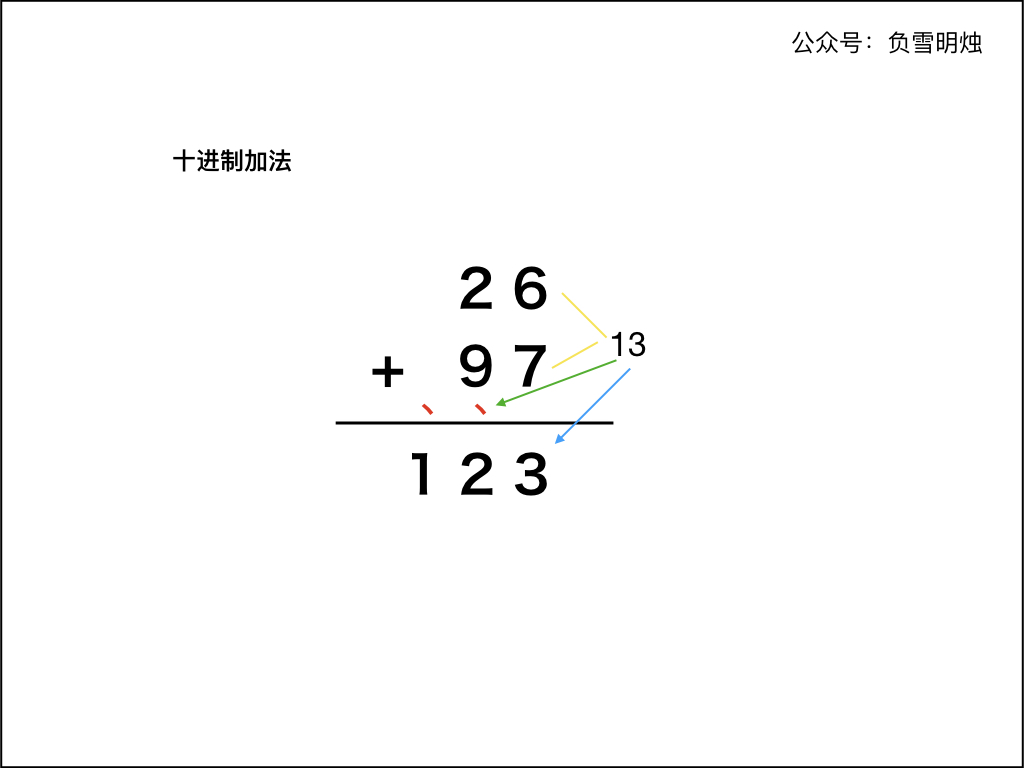# 989. Add to Array-Form of Integer 数组形式的整数加法

• 作者： 负雪明烛
• id： fuxuemingzhu
• 公众号：负雪明烛
• 本文关键词：LeetCode，力扣，算法，算法题，数组加法，两数之和，989，刷题群

@TOC

# # 题目描述

For a non-negative integer X, the array-form of X is an array of its digits in left to right order. For example, if X = 1231, then the array form is [1,2,3,1].

Given the array-form A of a non-negative integer X, return the array-form of the integer X+K.

Example 1:

Input: A = [1,2,0,0], K = 34
Output: [1,2,3,4]
Explanation: 1200 + 34 = 1234


Example 2:

Input: A = [2,7,4], K = 181
Output: [4,5,5]
Explanation: 274 + 181 = 455


Example 3:

Input: A = [2,1,5], K = 806
Output: [1,0,2,1]
Explanation: 215 + 806 = 1021


Example 4:

Input: A = [9,9,9,9,9,9,9,9,9,9], K = 1
Output: [1,0,0,0,0,0,0,0,0,0,0]
Explanation: 9999999999 + 1 = 10000000000


Note：

1. 1 <= A.length <= 10000
2. 0 <= A[i] <= 9
3. 0 <= K <= 10000
4. If A.length > 1, then A != 0

# # 解题方法

## # 前言

• 两个字符串形式的数字相加（第 415 题）
• 两个链表形式的数字相加（第 2 、445、369 题）
• 数组形式的数字相加（第 66 、989题）
• 两个二进制形式的数字相加（第 67 题）

## # 十进制加法1. 两个「加数」的右端对齐；
2. 从最右侧开始，从右向左依次计算对应的两位数字的和，如果有进位需要加上进位。如果和大于等于 10，则把和的个位数字计入结果，并向前面进位；
3. 重复步骤 2；
4. 当两个「加数」的每个位置都计算完成，如果最后仍有进位，需要把进位数字保留到计算结果中。

### # 在实现中需要注意的有：

1. 不可以把字符串表示的「加数」先转化成 int 型数字再求和，因为可能溢出
2. 两个「加数」的字符串长度可能不同；
3. 在最后，如果进位 carry 不为 0，那么最后需要计算进位
4. 注意 结果数字 是否为低位结果在前，根据题目要求判断最后是否要反转结果

## # 本题代码

### # 代码说明

1. while (p1 >= 0 || k != 0 || carry > 0)含义：
1. 字符串 num 和数字 k 只要有一个没遍历完，那么就继续遍历；
2. 如果字符串 num 和数字 k 都遍历完了，但是最后留下的进位 carry != 0，那么需要把进位也保留到结果中。
2. adder 的时候，如果字符串 num 和 数字 k 中有一个已经遍历完了（即 或者 ），则认为 numk 的对应位置是

### # 代码

Java 代码如下：

class Solution {
public List<Integer> addToArrayForm(int[] num, int k) {
List<Integer> res = new ArrayList<>(); // 返回结果
int p1 = num.length - 1; // 标记遍历到 num 的位置
int carry = 0; // 进位
while (p1 >= 0 || k != 0 || carry != 0) { // num 没遍历完，或 k 没遍历完，或进位不为 0
int adder1 = p1 >= 0 ? num[p1] : 0; // 当前 num 的取值
int adder2 = k % 10; // 当前 k 的位置，如果 k 已经是 0 那么 % 10 以后仍然是 0
carry = sum >= 10 ? 1 : 0; // 是否有进位
sum = sum >= 10 ? sum - 10 : sum; // 去除进位后留下的数字
p1 --; // 遍历到 num 的位置向左移动
k /= 10; // 取 k 的下一个位置的数字
}
Collections.reverse(res); // 把结果反转
return res;
}
}


C++ 代码如下：

class Solution {
public:
string addStrings(string num1, string num2) {
const int M = num1.size();
const int N = num2.size();
string res;
int p1 = M - 1;
int p2 = N - 1;
int carry = 0;
while (p1 >= 0 || p2 >= 0 || carry > 0) {
int cur1 = p1 >= 0 ? num1[p1] - '0' : 0;
int cur2 = p2 >= 0 ? num2[p2] - '0' : 0;
int sum = cur1 + cur2 + carry;
carry = sum >= 10 ? 1 : 0;
sum = sum >= 10 ? sum - 10 : sum;
res += to_string(sum);
p1 --;
p2 --;
}
reverse(res.begin(), res.end());
return res;
}
};


Python 代码如下：

class Solution(object):
p1 = len(num) - 1
carry = 0
res = []
while p1 >= 0 or k != 0 or carry > 0:
adder1 = num[p1] if p1 >= 0 else 0
carry = 1 if sum >= 10 else 0
sum = sum - 10 if sum >= 10 else sum
res.append(sum)
p1 -= 1
k //= 10
return res[::-1]


### # 复杂度分析

• 时间复杂度： 分别是 num 的长度 和 k 的位数；
• 空间复杂度：，只使用了常数的空间。

## # 总结

1. 求加法」系列题目都不难，其实就是 「列竖式」 计算。
2. 需要注意的是：
1. while循环结束条件；
2. 遍历两个「加数」不要越界；
3. 进位；
4. 最后的结果需要翻转。

# # 日期

2019 年 2 月 21 日 —— 一放假就再难抓紧了